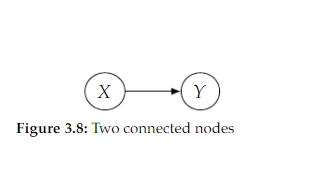Tags
#causality #has-images #statisticsQuestion
if the DAG were simply two connected nodes 𝑋 and 𝑌 as in Figure 3.8, the local Markov assumption would tell us that we can factorize 𝑃(𝑥, 𝑦) as 𝑃(𝑥)𝑃(𝑦|𝑥) , but it would also allow us to factorize 𝑃(𝑥, 𝑦) as 𝑃(𝑥)𝑃(𝑦) , meaning it allows distributions where 𝑋 and 𝑌 are independent. In contrast, the [...] assumption does not allow this additional independence
minimality

Tags
#causality #has-images #statisticsQuestion
if the DAG were simply two connected nodes 𝑋 and 𝑌 as in Figure 3.8, the local Markov assumption would tell us that we can factorize 𝑃(𝑥, 𝑦) as 𝑃(𝑥)𝑃(𝑦|𝑥) , but it would also allow us to factorize 𝑃(𝑥, 𝑦) as 𝑃(𝑥)𝑃(𝑦) , meaning it allows distributions where 𝑋 and 𝑌 are independent. In contrast, the [...] assumption does not allow this additional independence
?

Tags
#causality #has-images #statisticsQuestion
if the DAG were simply two connected nodes 𝑋 and 𝑌 as in Figure 3.8, the local Markov assumption would tell us that we can factorize 𝑃(𝑥, 𝑦) as 𝑃(𝑥)𝑃(𝑦|𝑥) , but it would also allow us to factorize 𝑃(𝑥, 𝑦) as 𝑃(𝑥)𝑃(𝑦) , meaning it allows distributions where 𝑋 and 𝑌 are independent. In contrast, the [...] assumption does not allow this additional independence
minimality
If you want to change selection, open original toplevel document below and click on "Move attachment"

#### Parent (intermediate) annotation

Open it
ll us that we can factorize 𝑃(𝑥, 𝑦) as 𝑃(𝑥)𝑃(𝑦|𝑥) , but it would also allow us to factorize 𝑃(𝑥, 𝑦) as 𝑃(𝑥)𝑃(𝑦) , meaning it allows distributions where 𝑋 and 𝑌 are independent. In contrast, the <span>minimality assumption does not allow this additional independence <span>

#### Original toplevel document (pdf)

owner: crocodile - (no access) - Introduction_to_Causal_Inference-Nov19_2020-Neal.pdf, p30

#### Summary

status measured difficulty not learned 37% [default] 0

No repetitions